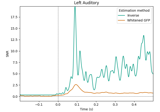# mne.viz.plot_snr_estimate#

mne.viz.plot_snr_estimate(evoked, inv, show=True, axes=None, verbose=None)[source]#

Plot a data SNR estimate.

Parameters:
evokedinstance of `Evoked`

The evoked instance. This should probably be baseline-corrected.

invinstance of `InverseOperator`

The minimum-norm inverse operator.

show`bool`

Show figure if True.

axesinstance of `Axes` | `None`

The axes to plot into.

New in version 0.21.0.

verbose

Control verbosity of the logging output. If `None`, use the default verbosity level. See the logging documentation and `mne.verbose()` for details. Should only be passed as a keyword argument.

Returns:
figinstance of `matplotlib.figure.Figure`

The figure object containing the plot.

Notes

The bluish green line is the SNR determined by the GFP of the whitened evoked data. The orange line is the SNR estimated based on the mismatch between the data and the data re-estimated from the regularized inverse.

New in version 0.9.0.

## Examples using `mne.viz.plot_snr_estimate`#Estimate data SNR using an inverse

Estimate data SNR using an inverse